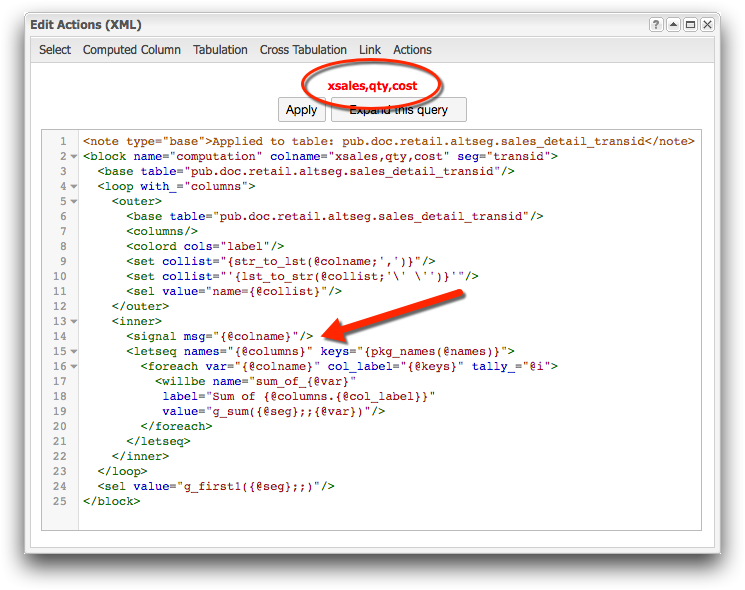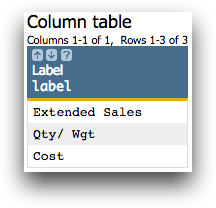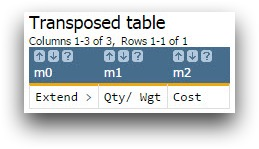# Determining the value of a variable

You can use a <signal> element to print out the value of a variable at a specified point in your query.

## Objective

The query you are writing keeps giving you an error at the same point each time you run it. You suspect the problem is that one if the variables is not receiving the expected value at that point in the query, and you need to find what value the variable is actually holding.

In this example a <foreach> loop performs multiple tabulations using two different variables. Upon executing the query, you receive the error Unequal length iterators in <foreach>. You aren't sure how long the iterators are or how to make them the same length.

## Solution

Here is the original code that is producing the error:

```<block name="computation" colname="xsales,qty,cost" seg="transid">
<base table="pub.doc.retail.altseg.sales_detail_transid"/>
<loop with_="columns">
<outer>
<base table="pub.doc.retail.altseg.sales_detail_transid"/>
<columns/>
<colord cols="label"/>
<set collist="{str_to_lst(@colname;',')}"/>
<set collist="'{lst_to_str(@collist;'\' \'')}'"/>
<sel value="name={@collist}"/>
</outer>
<inner>
<letseq names="{@columns}" keys="{pkg_names(@names)}">
<foreach var="{@colname}" col_label="{@keys}" tally_="@i">
<willbe name="sum_of_{@var}"
label="Sum of {@columns.{@col_label}}"
value="g_sum({@seg};;{@var})"/>
</foreach>
</letseq>
</inner>
</loop>
<sel value="g_first1({@seg};;)"/>
</block>```

First, you figure out what how many iterators are held in the variable colname.

```...
<inner>
<signal msg="{@colname}"/>
<letseq names="{@columns}" keys="{pkg_names(@names)}">
...```

Then, in a separate query, run the code in the outer to find out how many iterators are in the package columns.

```<block name="computation" colname="xsales,qty,cost" seg="transid">
<base table="pub.doc.retail.altseg.sales_detail_transid"/>
<columns/>
<colord cols="label"/>
<set collist="{str_to_lst(@colname;',')}"/>
<set collist="'{lst_to_str(@collist;'\' \'')}'"/>
<sel value="name={@collist}"/>
</block>```

And the final code with improvements is:

```<block name="computation" colname="xsales,qty,cost" seg="transid">
<base table="pub.doc.retail.altseg.sales_detail_transid"/>
<loop with_="columns">
<outer>
<base table="pub.doc.retail.altseg.sales_detail_transid"/>
<columns/>
<colord cols="label"/>
<set collist="{str_to_lst(@colname;',')}"/>
<set collist="'{lst_to_str(@collist;'\' \'')}'"/>
<sel value="name={@collist}"/>
<transpose/>
</outer>
<inner>
<letseq names="{@columns}" keys="{pkg_names(@names)}">
<foreach var="{@colname}" col_label="{@keys}" tally_="@i">
<willbe name="sum_of_{@var}" label="Sum of
{@columns.{@col_label}}" value="g_sum({@seg};;{@var})"/>
</foreach>
</letseq>
</inner>
</loop>
<sel value="g_first1({@seg};;)"/>
</block>```

## Discussion

To fix an error that has to do with the value(s) being held in a variable(s), it is helpful to use a <signal> message. Setting the message equal to the variable in question will return the value of that variable at the point in the code where the signal is placed. You know from the error message received that the problem lies with the values of the iterators in the <foreach> operation, colname and columns. From setting colname equal to the message, you will discover that there are three elements and therefore three iterators within the variable.However, you can't use the same method to discover the values held in columns because a signal message cannot display the value of a package. Instead, copy the code from the outer loop which is used to create the package and run it in a separate query. You also need to include the block so that you have the values of the variables used in this section of the code. After executing this code, you obtain the table that generates the package.The outer loop creates a package where each column name in the table is paired with the last item in the column. Therefore, this table generates a one item package. In order to create a three item package including each element in the table, you need to <transpose> this table.Now columns is a package that contains three pairs of values and when used with <letseq> in a <foreach> loop, creates three iterations.

## Further reading

If you would like to learn more about the functions and operations discussed in this recipe, click on the links below:

pkg(K;V)

<signal>